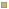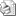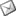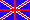HomeLogicsProposal for a new approach to Quantum Logic
Date Display
Wednesday, 28 September 2022
Last News
Correlated Article
History papers
Last twenty
 Proposal for a new approach to Quantum Logic## Proposal for a new approach to Quantum Logic##### In order to solve the problem of which is the real logic of Quantum Mechanics (QM), we suggest to study the mutual relationships between a physical theory (as a whole, that is both its experimental part and its theoretical part), its mathematics and its logic.

Abstract

In order to solve the problem of which is the real logic of Quantum Mechanics (QM), we suggest to study the mutual relationships between a physical theory (as a whole, that is both its experimental part and its theoretical part), its mathematics and its logic. That leads us to consider a more general problem than the problem considered by traditional studies on Quantum Logic (QL). These studies focus attention on the problem of the relationship between the experimental part of a physical theory and its mathematics only; from which logic is then derived. The new approach suggested by us generalises the interpretation that one of us (A. D.) successfully tested on the foundations of classical physical theories.

The foundations of a theory are interpreted in terms of two options on the kind of mathematics, either classical one or constructive one, and the kind of logic, either classical logic or intuitionistic logic. It is remarkable that each theory making use of intuitionistic logic – for instance, L. Carnot’s classical mechanics, S. Carnot’s thermodynamics, Lobacevskij’s non-Euclidean geometry - is organised in order to solve an universal problem (expressed by a double negated sentence), rather than in an entirely deductive way; hence, a non-classical logic does not belong exclusively to QM. From an historical and foundational analysis about QM, we conclude that in QM it is the law of double negation - and not modular law (introduced by Birkhoff and von Neumann without giving physical evidence to it) - which fails, i. e. which represents the true difference between classical logic and QL. We have looked for previous works on QL supporting our suggestion; in the past Destouches-Fevrier (1951), Fine (1974), Bell-Hallett (1981), Gauthier (1983), Adelman-Corbett (1995) already defended the relevance of double negation law for QL. According to our interpretation, QL has the same logic of a classical theory organised in a problematic manner, which is developed around a fundamental problem expressed by a double negated sentence; i.e., QM has to be referred to intuitionistic logic.

In particular, by following the founder of intuitionism, Brouwer, we reject the identification of intuitionistic logic with an entirely deductive system; rather, the logical system of QL as a theory has to be organised in such a way to put a crucial problem to be then solved. In agreement with this kind of organisation of both QM and QL, among several QM formulations we choose Heisenberg’s formulation, which was instead ignored by previous authors on QL. This formulation is aimed to solve the problem of quantum measurement, originating the uncertainty methodological principle.

Moreover, it is remarkable that it makes use of a mere operational mathematics (discrete matrices only). We interpret its characteristic arguing about its principal problem through double negated sentences; this arguing as a whole results to be a moving from arguments belonging to non-classical logic - expressed by double negated sentences - to the recognition of the suitable mathematics for QM formulation: in other words, QL governs the arguing stating the relationship between QM and mathematics. This point has been almost ignored by the traditional approaches to QL, which rather a priori follow a particular mathematics (commonly, Hilbert space). As a verification of our approach, we show that in each of two classical theories, i.e. Lobachevskij’s geometry and S. Carnot’s thermodynamics, the basic double negated sentences achieve the basic mathematical formulas of the subsequent development of the theory. We then show that two books on QM - by respectively T. F. Jordan (1985) and H. Weyl (1928) - formalised matrix mechanics in the same way.

Info

Drago A., Venezia A.: "“Proposal for a new approach to Quantum Logic”, in Proceedings Centenario Fisica Cuantica, Madrid 2000, in press